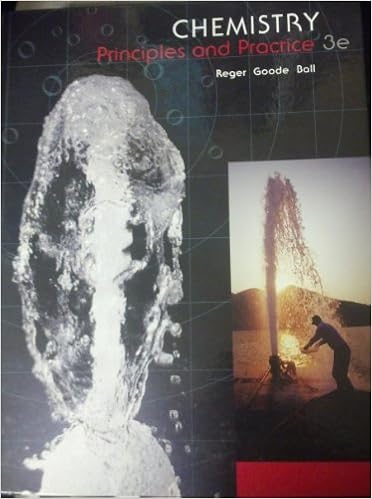# The total pressure exerted by a mixture of o 2 n 2

• Test Prep
• 12
• 100% (3) 3 out of 3 people found this document helpful

This preview shows page 6 - 9 out of 12 pages.

##### We have textbook solutions for you!
The document you are viewing contains questions related to this textbook.The document you are viewing contains questions related to this textbook.
Chapter 9 / Exercise 9.59
Chemistry: Principles and Practice
Reger ChemExpert Verified
24. The total pressure exerted by a mixture of O2, N2and He gases equals 10.0 atm. What is the partial pressure of O2if the partial pressure of N2is 5.0 atm and the partial pressure of He is 3.0 atm? a. 0.67 atm b. 2.0 atm c. 8.0 atm d. 12.0 atm e.
18.0 atm 25. What is the root mean square (rms) of H2(mm = 2.02 g/mol) gas at 30 K?
##### We have textbook solutions for you!
The document you are viewing contains questions related to this textbook.The document you are viewing contains questions related to this textbook.
Chapter 9 / Exercise 9.59
Chemistry: Principles and Practice
Reger ChemExpert Verified
CHEM111 – MYRICK FINAL EXAM, 7 Deember 2010 7 Questions from Chapter 7 26. What quantum number(s)is(are) required to completely describe an orbital, the region in space in which an electron has a high probability of being found? 1) Principal quantum number 2) Angular Momentum quantum number 3) Magnetic quantum number
27. At what wavelength (in nm) would emission occur for a hydrogen atom when an electron undergoes a transition from the n=6 to the n=4 level?
28. Which Orbital diagram for the ground state electronic configuration of a carbon atom is correct? (Remember Hund's Rule) 29. Only one set of quantum numbers listed below is a correct set. Which one? (n, l, ml, ms) a. 3, 2, 0, 1/2 b. 2, 2, -2, 1/2 c. 3, 3, -3, 1/2 d. 4, 4, -3, 1/2 e. none of these
CHEM111 – MYRICK FINAL EXAM, 7 Deember 2010 8 30. The threshold frequency for the photoelectric effect of a particular metal is 3.0 x 1014sec-1When this metal is illuminated by light with a frequency of 2.0 x 1014sec-1, which of the following is true? .
Questions from Chapter 8 31. Which series shown below is correctly arranged in order of increasing first ionization energy?
32. Which element would be expected to have the most favorable electron affinity?
F e. Be 33. What is the highest energy subshell that is occupied in the ground state of an atom of Bi (at. no. = 83)? a. 5p b. 5d c. 5f d. 6p e. none of these
34. The ground state electron configuration 1s22s22p63s23p2would be correct for which species listed below?
•••